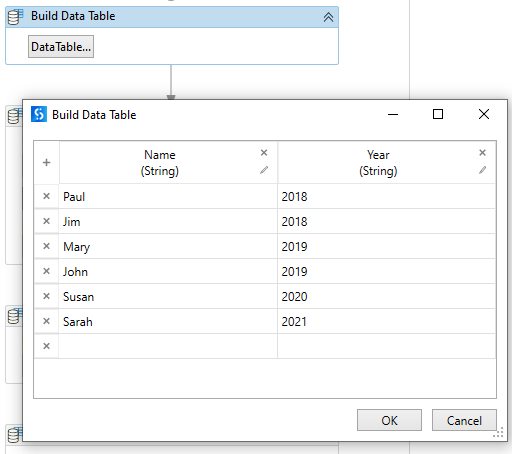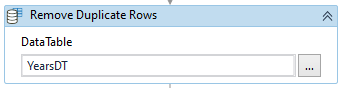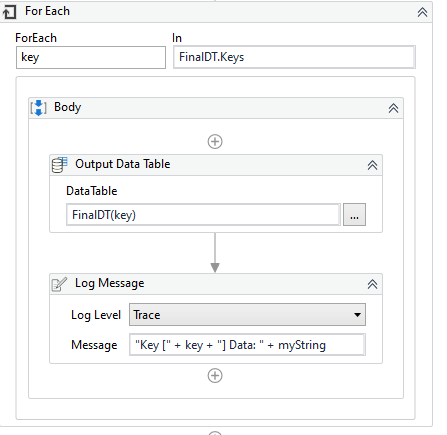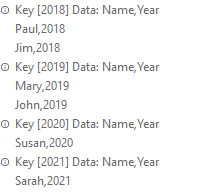# How to split a table in "n" tables each one containing a subset based on a single field of a record?

Hi,

at first glance, it appeared simple but I’m struggling.

Ok I have a table containing 1000 thousand records, one of the fields of the record is “year”, and what I need is to create as many tables as the numbers of years, ex. “2018”, “2019”…, each one containing only the records of that year.
It’s easy to do it knowing how many years you have and creating so many tables in advance to copy records after filtering. The problem is how to do it without knowing how many tables you need.
I used a list of list of data rows to group the records by year:
mylist = (From d In dtWork.AsEnumerable
Group d By k=d(1).toString.Trim Into grp=Group
Select grp.ToList).toList

Now if I want to create statically each list I only need to is:

dt1 = (From g In Groups
Where g.Any(Function ( r ) r(1).toString.Contains(“2018”))
Select g).SelectMany(Function (x) x).CopyToDataTable

But I want only populate data tables if I have records for that year so I have created a list of the data table and added the empty data tables to the collection then when I try to do the same using the table from the list like

list.Item(index) = (From g In Groups
Where g.Any(Function ( r ) r(1).toString.Contains(“2018”))
Select g).SelectMany(Function (x) x).CopyToDataTable

I got nothing.

I don’t know where is the mistake and may be there is an easy way to do all this.

Starting with SourceDT, use Filter Datatable into YearsDT to remove all columns except column with the year. Then use Remove Duplicate Rows on YearsDT. Now you have YearsDT as a datatable with only one column of unique year values.

Create FinalDT as dictionary(of string, datatable)

For Each Row through YearsDT
Inside the loop, use Filter Datatable with the source as SourceDT and the destination as FinalDT(CurrentRow(Year).ToString)

Now what you will have is a dictionary of datatables. The keys will be the years and the values will be the datatables filtered by year.

The variables:

Building a test datatable as SourceDT:Use Filter Datatable to remove all columns except Year, placing the result into YearsDT:

Remove Duplicate Rows from YearsDT:For Each through YearsDT and use Filter Datatable on Year into the FinalDT dictionary:

This last one is just to spit it all out into the Output pane:It looks like you are very close on the targeted solution

A trigger for grouping data on year

we can put it within the LINQ

Assign Activity
LHS TableList | DataType: List(Of DataTable)
RHS:

``````(From d In dtWork.AsEnumerable
Group d By k=d(1).toString.Trim Into grp=Group
Select grp.CopyToDataTable).toList
``````

each item within the TableList will be a DataTable holding all rows for a particular Year. It is dynamic and requires no precalculations of existing years

Also have a look here:

A NoN-LINQ Approach is described as well

On that flow just add the datatable from the filter Data Table activity to a list (e.g. TableList)

Thanks very much!
I tried the solution of creating a list of data tables and then looping through the list-saving tables to excel files.

But I also found a linq solution very useful and elegant for my purpose this one that I had to modify a bit

(From d In dtWork.AsEnumerable
Group d By k=d(“Year”).toString.Trim Into grp=Group
Let cn = String.Join(“;”, grp.Select(Function (rn) rn(“CaseName”).toString).toArray)
Let ra = New Object(){k, cn}